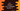# C++ program to check if a number is divisible by 5 and 11## C++ program to check if a number is divisible by 5 and 11:

In this post, we will learn how to check if a number is divisible by 5 and 11 or not. This program will take one number as input from the user and it will print the result, i.e. if it is divisible by both 5 and 11 or not.

With this program, you will learn how to take user inputs in C++, how to print result on console, how to use modulo operator and how to use if-else blocks in C++.

### Algorithm:

We will follow the below algorithm:

• Take the number as input from the user.
• Check if it is divisible by 5 and 11 or not.
• Print one message to the user.

### Modulo operator:

The modulo operator is used to get the remainder of a division. % is used for modulo operator. For example, if x and y are two numbers, x%y will give the remainder of x/y, i.e. the remainder we will get if x is divided by y.

For example, 10%2 is 0 and 11%2 is 1.

## Method 1: C++ program to check if a number is divisible by 5 and 11 by using a if-else block:

Below is the complete program:

``````#include <iostream>

using namespace std;

int main()
{
int no;

cout << "Enter a number: " << endl;
cin >> no;

if (no % 5 == 0 && no % 11 == 0)
{
cout << "It is divisible by 5 and 11" << endl;
}
else
{
cout << "It is not divisible by 5 and 11" << endl;
}
}``````

Here,

• no is an integer variable to hold the user input number.
• Using cout and cin, we are reading the number as input from the user.
• The if block checks if this number is divisible by 5 and 11 or not.
• If yes, it runs the body of the if block. Else, it runs the body of the else block.

If you run this program, it will print output as like below:

``````Enter a number:
55
It is divisible by 5 and 11

Enter a number:
11
It is not divisible by 5 and 11``````

## Method 2: C++ program to check if a number is divisible by 5 and 11 by using a ternary or conditional operator:

We can also use conditional or ternary operator to write this program. The conditional or ternary operator is similar to if-else block, but we can use it to write the same check in just one line.

It is defined as like below:

``exp ? code1 : code2``

It will execute code1 if exp is true. Else, it will execute code2.

Let’s write down the complete program:

``````#include <iostream>

using namespace std;

int main()
{
int no;

cout << "Enter a number: " << endl;
cin >> no;

(no % 5 == 0 && no % 11 == 0) ? cout << "It is divisible by 5 and 11" << endl : cout << "It is not divisible by 5 and 11" << endl;
}``````

The expression is (no % 5 == 0 && no % 11 == 0) and it is checking if the number is divisible by 5 and 11 or not. It is printing one message based on its result.

If you run this program, it will print similar output.